When going over Gradient boosting I got confused why there was a discrepancy between the residual and the weak learner. So here is an attempt in clearing up that confusion.

We assume we are given an imperfect model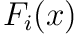and we want to improve it by only by additive changes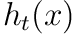, i.e.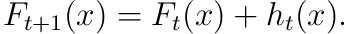If we chose the perfectthis would simply be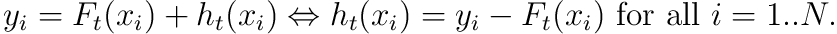For the square lossthis turns out to simply be equivalent to the negative gradient of the loss, i.e.Now the crucial observation is that the class of weak learners that we allow are in general not expressive enough to capture the residualperfectly. So instead we choose the model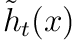which minimizes this difference with this residual.

The algorithm then proceeds by updating the model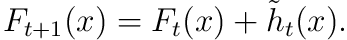It is thus an iterative scheme that at every step more generally uses the negative gradient of the loss to build the estimator.